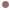Autres Articles de :
Algorithms

# Estimating Shannon Entropy from Recurrence Plots

Christophe LETELLIER
18/05/2009

Recurrence plots were first introduced to quantify the recurrence properties of chaotic dynamics . A few years later, the recurrence quantification analysis was introduced to transform graphical representations into statistical analysis . Among the different measures introduced, a Shannon entropy was found to be correlated with the inverse of the largest Lyapunov exponent. The discrepancy between this and the usual interpretation of a Shannon entropy has been solved by replacing the probabilities’s used in the entropy formulaThe key point is to replacewith the number of diagonal segments of non-recurrent points (made of white dots) divided by the number of recurrent points . Indeed, a white dot representing a non-recurrent point is nothing more than a signature of complexity within the data. With this definition,increases as the bifurcation parameter increases (as shown for the Logistic map in Fig. 1b). There is a one-to-one correspondence between the new definition ofand the positive largest Lyapunov exponent.Fig. 1 : Comparison between the Largest Lyapunov Exponent and the Shannon Entropy.Estimating the Shannon entropy for the Logistic map

The algorithm provided here computed the Shannon Entropy from Recurrence Plots for the Logistic map versus parameter. It produced Fig. 1. It is also possible to add noise.A second Fortran code is provided. It computes the Shannon entropy using a recurrence plot from a data file. you have to specify the number of data point (Npoint). Your data file is expected to have a single column. The code returns

• the recurrence rate ;
• the "determinism" rate ;
• the Shannon entropy.

Be aware that the so-called "determinism" rate does not provide in fact a determinism rate for the simple reason that, for instance, it is equal to 0.82 when estimated from a time series produced by the Logistic map with, a signal which is 100% deterministic ! So, please, interpret with great care this rate. Note also that, for flows, it is definitely better to compute recurrence plots from a "discrete" time series recorded in the Poincaré section of the attractor than from a "continuous" time series. This is discussed in Ref. .Fortran code for the Shannon entropy from data file

 J.-P. Eckmann, S. Oliffson Kamphorst & D. Ruelle, Recurrence Plots of Dynamical Systems, Europhysics Letters, 4, 973-977, 1987.

 L. L. Trulla, A. Giuliani, J. P. Zbilut & C. L. Webber Jr., Recurrence quantification analysis of the logistic equation with transients, Physics Letters A, 223 (4), 255-260, 1996.

 This latter detail was missing in the definition provided in C. Letellier, Estimating the Shannon entropy : recurrence plots versus symbolic dynamics, Physical Review Letters, 96, 254102, 2006.

### Documents

Estimating the Shannon entropy for the Logistic mapZip · 1.5 ko
3948 - 12/12/19

Fortran code for the Shannon entropy from data fileZip · 1.2 ko
5929 - 12/12/19